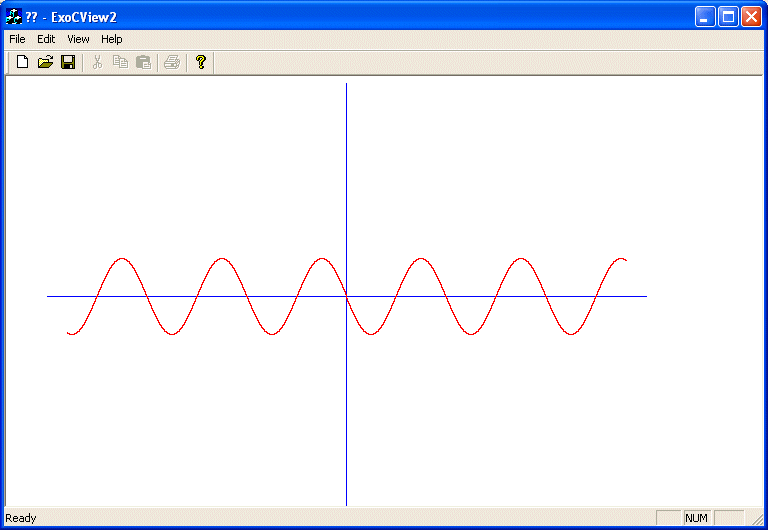YOU CAN CODE!With The Case Of UCanCode.net  Release The Power OF  Visual C++ ! Home |  Products | Purchase |  Support | Downloads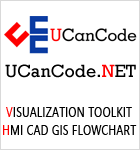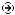Download EvaluationPricing & Purchase?E-XD++Visual C++/ MFC ProductsOverviewElectronic Form SolutionVisualization & HMI SolutionPower system HMI SolutionBar code labeling SolutionWorkflow SolutionCoal industry HMI SolutionInstrumentation Gauge SolutionReport Printing SolutionGraphical modeling SolutionGIS mapping solutionVisio graphics solutionIndustrial monitoring SolutionFlowchart and diagramming SolutionOrganization Diagram SolutionGraphic editor Source CodeUML drawing editor Source CodeMap Diagramming SolutionArchitectural Graphic Drawing SolutionRequest EvaluationActiveX COM ProductsOverviewTechnical Support# Get Ready to Unleash the Power of UCanCode .NET

UCanCode Software focuses on general application software development. We provide complete solution for developers. No matter you want to develop a simple database workflow application, or an large flow/diagram based system, our product will provide a complete solution for you. Our product had been used by hundreds of top companies around the world!

"100% source code provided! Free you from not daring to use components because of unable to master the key technology of components!"

The GDI Coordinate Systems, SetViewportOrg, SetWindowExt, SetViewportExt, SetMapMode

The Default Coordinate System

When drawing on Microsoft Windows, the coordinates of the drawing area are located on the upper-left corner of the screen. Everything positioned on the screen takes its reference on that point. That point can be illustrated in a Cartesian coordinate system as (0,0) where the horizontal axis moves from (0,0) to the right and the vertical axis moves from (0,0) down:This starting origin is only the default coordinate system of the operating system. Therefore, if you draw a shape with the following call, Ellipse(-100, -100, 100, 100), you would get a circle whose center is positioned on the top-left corner of the screen. In this case, only the lower-right 3/4 of the circle would be seen:

```void CExoDraw1View::OnPaint()
{
CPaintDC dc(this); // device context for painting

CPen PenBlue;

// Blue solid pen width = 1
PenBlue.CreatePen(PS_SOLID, 1, RGB(0, 12, 255));

dc.SelectObject(&pPen);
dc.Ellipse(-100, -100, 100, 100);
}```In the same way, you can draw any geometric or non-geometric figure you want, using one of the CPaintDC methods or creating methods of your choice. For example, the following code draws a vertical and a horizontal lines that cross each other in the center middle of the form:
 ```void CExoDraw1View::OnPaint() { CPaintDC dc(this); // device context for painting CRect Recto; CPen PenBlue; PenBlue.CreatePen(PS_SOLID, 1, RGB(0, 12, 255)); dc.SelectObject(&PenBlue); dc.Ellipse(-100, -100, 100, 100); CPen PenBlack; PenBlack.CreatePen(PS_SOLID, 1, BLACK_PEN); dc.SelectObject(&PenBlack); // Retrive the size of the drawing area GetClientRect(&Recto); dc.MoveTo(Recto.Width() / 2, 0); dc.LineTo(Recto.Width() / 2, Recto.Height()); dc.MoveTo(0, Recto.Height() / 2); dc.LineTo(Recto.Width(), Recto.Height() / 2); }```Changing the GDI Coordinate System
 As seen above, the default coordinate system has its origin set on the top-left section of the screen. The horizontal axis moves positively from the origin to the right direction. The vertical axis moves from the origin to the bottom direction. To illustrate this, let's draw a circle with a radius whose center is at the origin (0, 0) with a radius of 50 units. Let's also draw a line from the origin (0, 0)  to (100, 100):
 ```void CExoDraw1View::OnPaint() { CPaintDC dc(this); // device context for painting // A circle whose center is at the origin (0, 0) dc.Ellipse(-50, -50, 50, 50); // A line that starts at (0, 0) and ends at (100, 100) dc.MoveTo(0, 0); dc.LineTo(100, 100); }```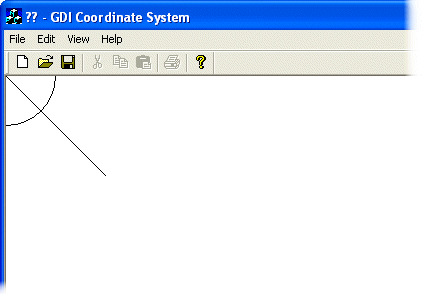This default origin is fine for most, if not all regular, operations performed on graphics applications. For example, most graphics application, including Paint Shop Pro use this origin. Sometimes, you will need to control the position of the origin of the coordinate system. For example, most CAD applications, including AutoCAD, allow the user to set this origin. The MFC provides various functions to deal with the coordinates positions and extents of the drawing area, including functions used to set the origin of the coordinate system anywhere you want on the screen. Since you are drawing on a device context, all you need to do is simply call the CDC::SetViewportOrg() method. It is overloaded with two versions, which allow you to use either the X and the Y coordinates or a defined point. The syntaxes of this method are:
 `SetViewportOrg(int X, int Y);` `SetViewportOrg(CPoint Pt);`
 When calling this function, simply specify where you want the new origin to be. If using the second version, the argument can be a Win32 POINT structure or an MFC CPoint class. To see the effect of this function, let's move the origin 200 units in the positive direction of the X axis and 150 units in the positive direction of the vertical axis without changing the circle and the line. Our OnPaint event would look like this:
 ```void CExoDraw1View::OnPaint() { CPaintDC dc(this); // device context for painting dc.SetViewportOrg(200, 150); // A circle whose center is at the origin (0, 0) dc.Ellipse(-50, -50, 50, 50); // A line that starts at (0, 0) and ends at (100, 100) dc.MoveTo(0, 0); dc.LineTo(100, 100); }```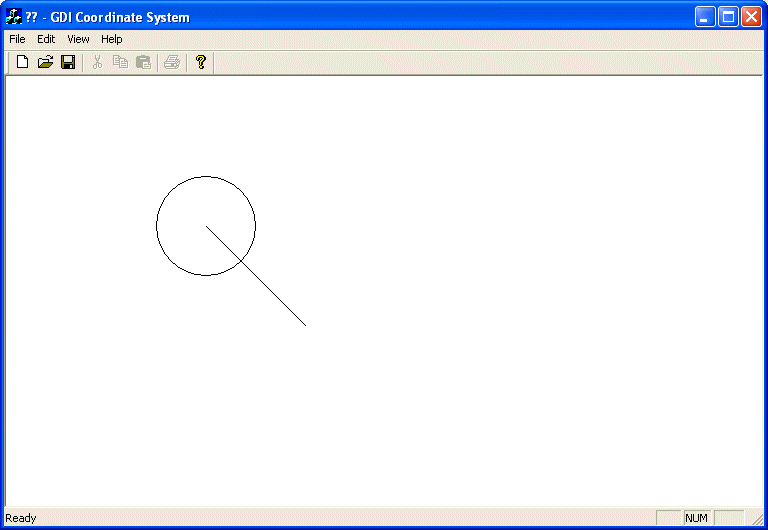Note that you can also position the origin relative to the size of the client area. Here is an example:
 ```void CExoDraw1View::OnPaint() { CPaintDC dc(this); // device context for painting CRect Recto; // Retrieve the size of the drawing area GetClientRect(&Recto); dc.SetViewportOrg(Recto.Width() / 2, Recto.Height() / 2); // A circle whose center is at the origin (0, 0) dc.Ellipse(-50, -50, 50, 50); // A line that starts at (0, 0) and ends at (100, 100) dc.MoveTo(0, 0); dc.LineTo(100, 100); }```Now that we know how to control the origin, we will position it at a fixed point, 380 units to the right and 220 units down. We can also easily draw the (Cartesian) axes now:
 ```void CExoDraw1View::OnPaint() { CPaintDC dc(this); // device context for painting CRect Recto; dc.SetViewportOrg(380, 220); // Use a red pen CPen PenRed(PS_SOLID, 1, RGB(255, 0, 0)); dc.SelectObject(PenRed); // A circle whose center is at the origin (0, 0) dc.Ellipse(-100, -100, 100, 100); // Use a blue pen CPen PenBlue(PS_SOLID, 1, RGB(0, 0, 255)); dc.SelectObject(PenBlue); // Horizontal axis dc.MoveTo(-380, 0); dc.LineTo(380, 0); // Vertical axis dc.MoveTo(0, -220); dc.LineTo(0, 220); }```As seen already, the SetViewportOrg() method can be used to change the origin of the device context. It also uses an orientation of axes so that the horizontal axis moves positively from (0, 0) to the right. The vertical axis moves positively from (0, 0) down.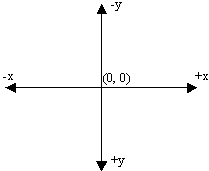To illustrate this, let's draw an orange line at 45° from the origin:
 ```void CExoDraw1View::OnPaint() { CPaintDC dc(this); // device context for painting dc.SetViewportOrg(380, 220); // Use a red pen CPen PenRed(PS_SOLID, 1, RGB(255, 0, 0)); dc.SelectObject(PenRed); // A circle whose center is at the origin (0, 0) dc.Ellipse(-100, -100, 100, 100); // Use a blue pen CPen PenBlue(PS_SOLID, 1, RGB(0, 0, 255)); dc.SelectObject(PenBlue); // Horizontal axis dc.MoveTo(-380, 0); dc.LineTo(380, 0); // Vertical axis dc.MoveTo(0, -220); dc.LineTo(0, 220); // An orange pen CPen PenOrange(PS_SOLID, 1, RGB(255, 128, 0)); dc.SelectObject(PenOrange); // A diagonal line at 45 degrees dc.MoveTo(0, 0); dc.LineTo(120, 120); }```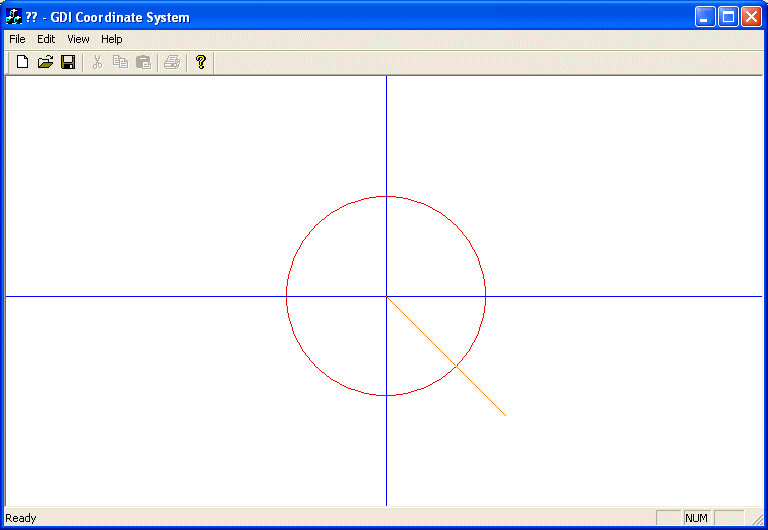As you can see, our line is not at 45º. Instead of being in the first quadrant, it is in the fourth. This is due to the default orientation of the coordinate system.
 The Map Modes
 To control the orientation of the axes of the device context, you use a method of the CDC class called SetMapMode(). Its syntax is: `int SetMapMode(int nMapMode);` As you are about to see, this method can be used to do two things, depending on the value of the argument. It can control the orientation of the coordinate system you want to use for your application. It also helps with the unit system you would prefer to use.  The argument of this method is a constant integer that species the mapping mode used. The possible values are MM_TEXT, MM_LOENGLISH, MM_HIENGLISH, MM_ANISOTROPIC, MM_HIMETRIC, MM_ISOTROPIC, MM_LOMETRIC, and MM_TWIPS.
 The default map mode used is the MM_TEXT. In other words, if you don't specify another, this is the one your application would use. With this map mode, the dimensions or measurements you specify in your CDC methods are respected and kept "as is". Also, the axes are oriented so the horizontal axis moves from (0, 0) to the right and the vertical axis moves from (0, 0) down. For example, the above OnPaint event can be re-written as follows and would produce the same result:
 ```void CExoDraw1View::OnPaint() { CPaintDC dc(this); // device context for painting dc.SetMapMode(MM_TEXT); dc.SetViewportOrg(380, 220); // Use a red pen CPen PenRed(PS_SOLID, 1, RGB(255, 0, 0)); dc.SelectObject(PenRed); // A circle whose center is at the origin (0, 0) dc.Ellipse(-100, -100, 100, 100); // Use a blue pen CPen PenBlue(PS_SOLID, 1, RGB(0, 0, 255)); dc.SelectObject(PenBlue); // Horizontal axis dc.MoveTo(-380, 0); dc.LineTo(380, 0); // Vertical axis dc.MoveTo(0, -220); dc.LineTo(0, 220); // An orange pen CPen PenOrange(PS_SOLID, 1, RGB(255, 128, 0)); dc.SelectObject(PenOrange); // A diagonal line at 45 degrees dc.MoveTo(0, 0); dc.LineTo(120, 120); }```

The result is the same, as if no map mode was specified:The MM_LOENGLISH, like some of the other map modes (excluding MM_TEXT as seen above), performs two actions. It changes the orientation of the vertical axis: the positive y axis would move from (0, 0) up:Also, each unit of measure is multiplied by 0.01 inch, which means each unit you provide is divided by 100 (unit/100). This also means that the units are reduced from their stated measures by a 100th. Observe the effect of the MM_LOENGLISH map mode on the above OnPaint() event :
 ```void CExoDraw1View::OnPaint() { CPaintDC dc(this); // device context for painting dc.SetMapMode(MM_LOENGLISH); dc.SetViewportOrg(380, 220); . . . }```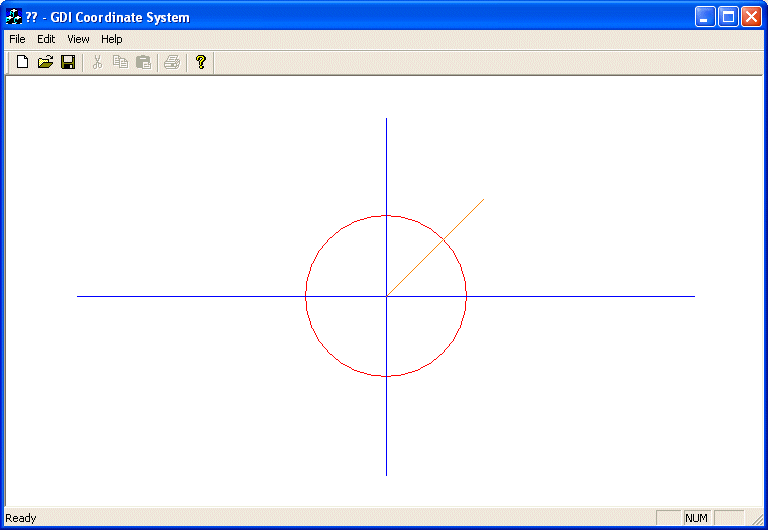As you can see, now the lines are drawn respecting the positive and the negative orientations of the axes, fulfilling the requirements of a Cartesian coordinate system. At the same time, the lengths we used have been reduced: the circle is smaller and the lines are shorter. Like the MM_LOENGLISH map mode, the MM_HIENGLISH sets the orientation so the vertical axis moves from (0, 0) up. Unlike the MM_LOENGLISH, the MM_HIENGLISH map mode reduces each unit by a factor of 0.001 inch. This means that each unit is divided by 1000 (1/1000 = 1000th) which is significant and can change the display of a drawing. Here is its effect:
 ```void CExoDraw1View::OnPaint() { CPaintDC dc(this); // device context for painting dc.SetMapMode(MM_HIENGLISH); dc.SetViewportOrg(380, 220); . . . Same as previous }```Notice that we are still using the same dimensions for our lines and circle. The MM_LOMETRIC map mode uses the same axes orientation as the previous two modes. By contrast, the MM_LOMETRIC multiplies each unit by 0.1 millimeter. This means that each unit is reduced by 10%. Here is an example:
 ```void CExoDraw1View::OnPaint() { CPaintDC dc(this); // device context for painting dc.SetMapMode(MM_LOMETRIC); dc.SetViewportOrg(380, 220); . . . }```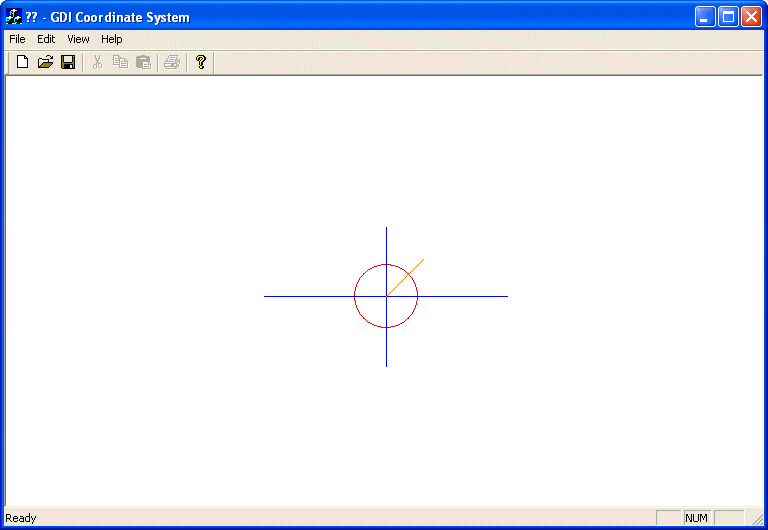The MM_HIMETRIC map mode uses the same axes of orientation as the above three modes. Its units are gotten by multiplying each of the given units by 0.01 millimeter. Here is an example:
 ```void CExoDraw1View::OnPaint() { CPaintDC dc(this); // device context for painting dc.SetMapMode(MM_HIMETRIC); dc.SetViewportOrg(380, 220); . . . Same as previous }```The MM_TWIPS map mode divides each logical unit by 20. Actually a twip is equivalent to 1/1440 inch. Besides this unit conversion, the axes are oriented so the horizontal axis moves from the origin (0, 0) to the right while the vertical axis moves from the origin (0, 0) up. Here is an example:
 ```void CExoDraw1View::OnPaint() { CPaintDC dc(this); // device context for painting CRect Recto; dc.SetMapMode(MM_TWIPS); dc.SetViewportOrg(380, 220); . . . }```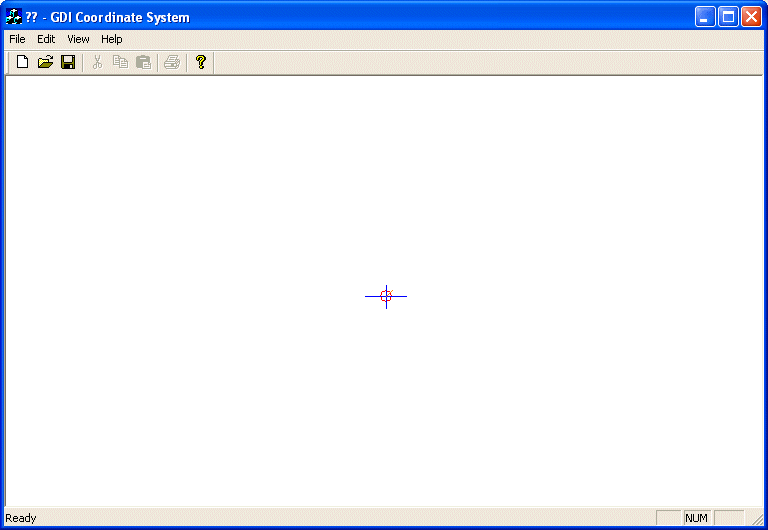Customizing the Unit and Coordinate Systems
 The map modes we have used so far allowed us to select the orientation of the axes, especially the y axis. Furthermore, we couldn't influence any conversion unit for the dimensions we specified on our drawings. This is because each one of these mapping modes (MM_TEXT, MM_HIENGLISH, MM_LOENGLISH, MM_HIMETRIC, MM_LOMETRIC, and MM_TWIPS) has a fixed set of attributes such as the orientation of its axes and the conversion used on the provided dimensions. What if you want to control the orientation of axes and/or the conversion applied on the dimensions you provide in your drawing (have you ever used AutoCAD?). Consider the following OnPaint() event. It draws a 200x200 pixels square with a red border and an aqua background. The square starts at 100x100 pixels on the negative sides of both axes and it continues 100x100 pixels on the positive sides of both axes. For better illustration, the event also draws a diagonal line at 45º starting at the origin (0, 0):
 ```void CExoDraw1View::OnPaint() { CPaintDC dc(this); // device context for painting CPen PenRed(PS_SOLID, 1, RGB(255, 0, 0)); CBrush BrushAqua(RGB(0, 255, 255)); dc.SelectObject(PenRed); dc.SelectObject(BrushAqua); // Draw a square with a red border and an aqua background dc.Rectangle(-100, -100, 100, 100); CPen BluePen(PS_SOLID, 1, RGB(0, 0, 255)); dc.SelectObject(BluePen); // Diagonal line at 45 degrees starting at the origin (0, 0) dc.MoveTo(0, 0); dc.LineTo(200, 200); }```

This would produce:As you can see, we get only the the lower-right 3/4 portion of the square and the line is pointing in the 3 to 6 quadrant of a clock . Imagine that you want the origin (0, 0) to be positioned in the center middle of the form, or to be more precise, to position the origin at (340, 220). We saw already that you could use the CDC::SetViewportOrg() method (keep in mind that this method only changes the origin of the coordinate system; it doesn't influence the orientation of axes nor does it control the units or dimensions) to specify the origin. Here is an example (we are not specifying the map mode because MM_TEXT can be used for us as the default):
 ```void CExoDraw1View::OnPaint() { CPaintDC dc(this); // device context for painting dc.SetViewportOrg(340, 220); CPen PenRed(PS_SOLID, 1, RGB(255, 0, 0)); CBrush BrushAqua(RGB(0, 255, 255)); dc.SelectObject(PenRed); dc.SelectObject(BrushAqua); // Draw a square with a red border and an aqua background dc.Rectangle(-100, -100, 100, 100); CPen BluePen(PS_SOLID, 1, RGB(0, 0, 255)); dc.SelectObject(BluePen); // Diagonal line at 45 degrees starting at the origin (0, 0) dc.MoveTo(0, 0); dc.LineTo(200, 200); }```

This would produce:To control your own unit system, the orientation of the axes or how the application converts the units used on your application, use either the MM_ISOTROPIC or the MM_ANISOTROPIC map modes. The first thing you should do is to call the CDC::SetMapMode() method and specify one of these two constants (either MM_ISOTROPIC or MM_ANISOTROPIC). Here is an example:
 ```void CExoDraw1View::OnPaint() { CPaintDC dc(this); // device context for painting dc.SetMapMode(MM_ISOTROPIC); dc.SetViewportOrg(340, 220); CPen PenRed(PS_SOLID, 1, RGB(255, 0, 0)); CBrush BrushAqua(RGB(0, 255, 255)); dc.SelectObject(PenRed); dc.SelectObject(BrushAqua); // Draw a square with a red border and an aqua background dc.Rectangle(-100, -100, 100, 100); CPen BluePen(PS_SOLID, 1, RGB(0, 0, 255)); dc.SelectObject(BluePen); // Diagonal line at 45 degrees starting at the origin (0, 0) dc.MoveTo(0, 0); dc.LineTo(200, 200); }```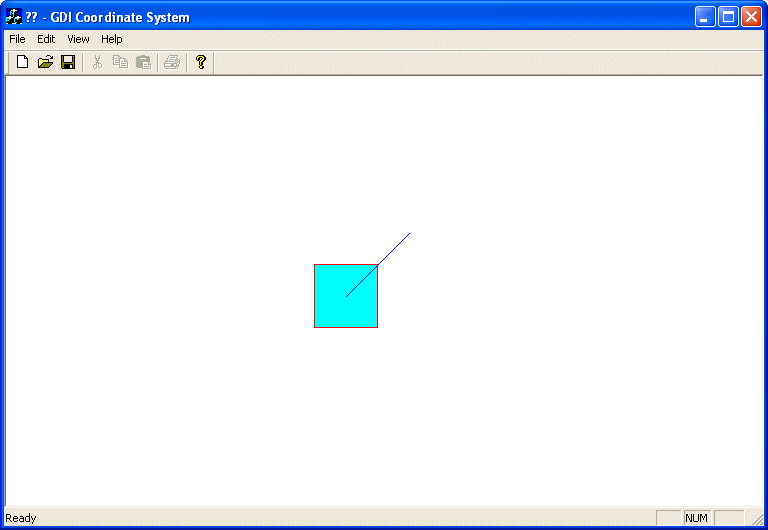Don't rely on the above picture, after calling the CDC::SetMapMode() method with MM_ISOTROPIC (or MM_ANISOTROPIC) as argument, you are not supposed to stop there. The purpose of these two map modes is to let you control the orientation of the axes and the conversion of the units. The difference between both map modes is that, when using the MM_ISOTROPIC map mode, one unit in the horizontal axis is equivalent to one unit in the vertical axis. This is not the case for the MM_ANISOTROPIC map mode which allows you to control however the units should be converted on each individual axis. Therefore, after calling SetMapMode() and specifying the MM_ISOTROPIC (or MM_ANISOTROPIC),  you must call the CDC:SetWindowExt() method. This method specifies how much each new unit will be multiplied by the old or default unit system. The CDC::SetWindowExt() method comes in two versions with the following syntaxes: ```CSize SetWindowExt(int cx, int cy); CSize SetWindowExt(SIZE size);``` If using the first version, the first argument to this method, cx, specifies the logical conversion multiplier used for each unit on the horizontal axis. The second argument, cy, specifies the logical conversion multiplier used for each unit on the vertical axis. The second version of the method can be used if you know the desired logical width and height as a SIZE object. Here is an example:
 ```void CExoDraw1View::OnPaint() { CPaintDC dc(this); // device context for painting dc.SetMapMode(MM_ISOTROPIC); dc.SetViewportOrg(340, 220); dc.SetWindowExt(480, 480); CPen PenRed(PS_SOLID, 1, RGB(255, 0, 0)); CBrush BrushAqua(RGB(0, 255, 255)); dc.SelectObject(PenRed); dc.SelectObject(BrushAqua); // Draw a square with a red border and an aqua background dc.Rectangle(-100, -100, 100, 100); CPen BluePen(PS_SOLID, 1, RGB(0, 0, 255)); dc.SelectObject(BluePen); // Diagonal line at 45 degrees starting at the origin (0, 0) dc.MoveTo(0, 0); dc.LineTo(200, 200); }```

This would produce: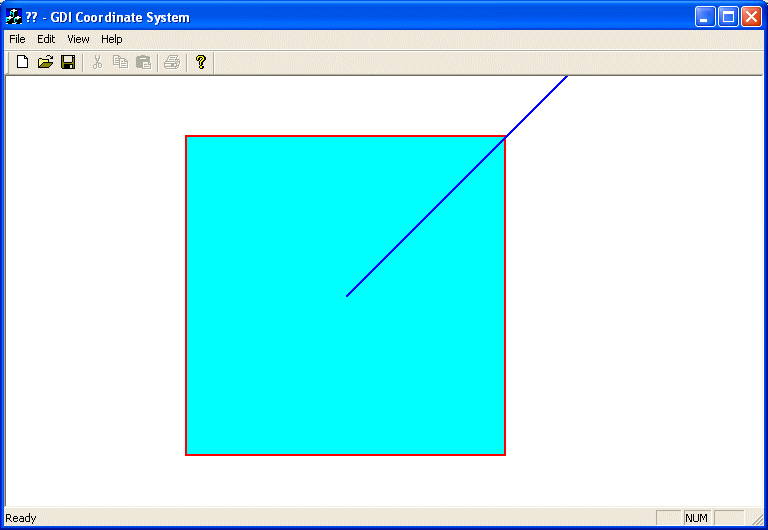After calling the SetWindowExt() function, you should call the SetViewportExt() function. Its job is to specify the horizontal and vertical units of the device context being used. It comes in two flavors with the following syntaxes: ```CSize SetViewportExt(int cx, int cy); CSize SetViewportExt(SIZE size);``` To use the first version of this function, you must provide the units of device conversion as cx for the horizontal axis and as cy for the vertical axis. If you know the size as a width/height combination of the device unit conversion, you can use the second version of the method and supply this size argument. Here is an example:
 ```void CExoDraw1View::OnPaint() { CPaintDC dc(this); // device context for painting dc.SetMapMode(MM_ISOTROPIC); dc.SetViewportOrg(340, 220); dc.SetWindowExt(480, 480); dc.SetViewportExt(440, -680); CPen PenRed(PS_SOLID, 1, RGB(255, 0, 0)); CBrush BrushAqua(RGB(0, 255, 255)); dc.SelectObject(PenRed); dc.SelectObject(BrushAqua); // Draw a square with a red border and an aqua background dc.Rectangle(-100, -100, 100, 100); CPen BluePen(PS_SOLID, 1, RGB(0, 0, 255)); dc.SelectObject(BluePen); // Diagonal line at 45 degrees starting at the origin (0, 0) dc.MoveTo(0, 0); dc.LineTo(200, 200); }```

This would produce: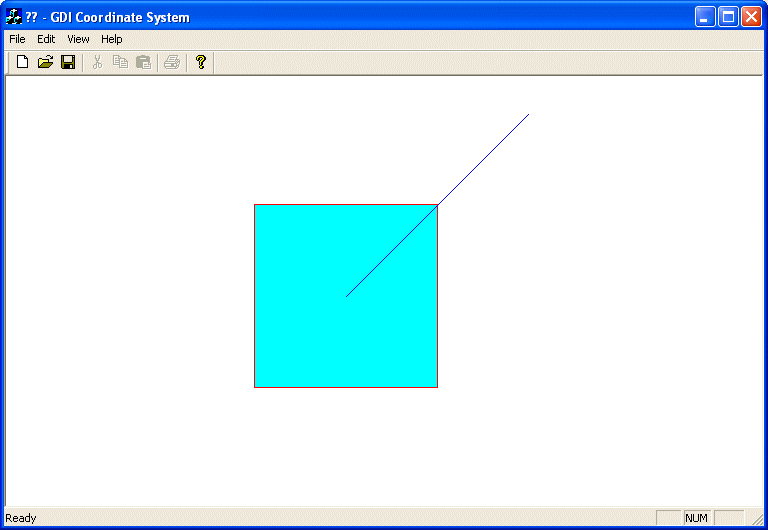Example drawing axes orientation arrows: ```void CExoDraw1View::OnPaint() { CPaintDC dc(this); // device context for painting CBrush bgBrush(BLACK_BRUSH); dc.SelectObject(bgBrush); dc.Rectangle(Recto); dc.SetMapMode(MM_ISOTROPIC); dc.SetViewportOrg(0, 440); dc.SetWindowExt(480, 480); dc.SetViewportExt(440, -680); CPen PenWhite(PS_SOLID, 1, RGB(255, 255, 255)); dc.SelectObject(PenWhite); dc.MoveTo(21, 20); dc.LineTo(21, 75); // Up arrow dc.MoveTo(16, 75); dc.LineTo(21, 90); dc.LineTo(26, 75); dc.LineTo(16, 75); dc.MoveTo(21, 22); dc.LineTo(75, 22); // Right arrow dc.MoveTo(75, 17); dc.LineTo(90, 22); dc.LineTo(75, 27); dc.LineTo(75, 17); dc.SetBkMode(TRANSPARENT); dc.SetTextColor(RGB(255, 255, 255)); dc.TextOut(16, 114, 'Y'); dc.TextOut(100, 32, 'X'); dc.Rectangle(15, 15, 30, 30); }```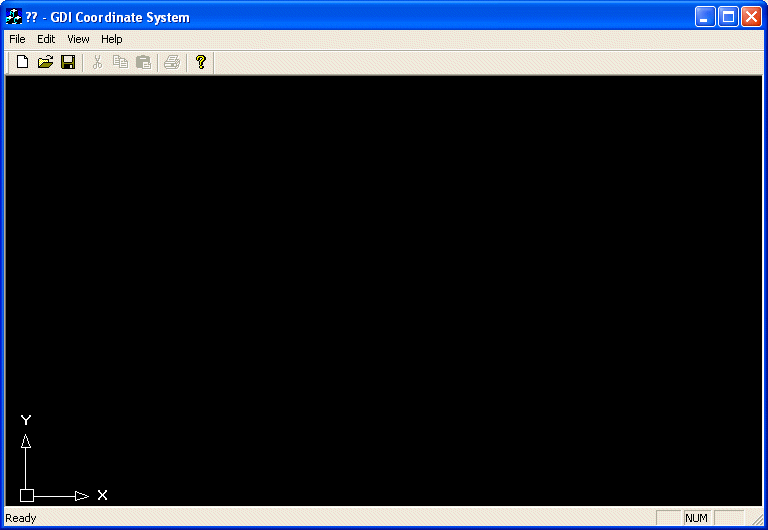Line Grid ```void CExoDraw1View::OnPaint() { CPaintDC dc(this); // device context for painting CRect Recto; GetClientRect(&Recto); CBrush bgBrush(BLACK_BRUSH); dc.SelectObject(bgBrush); dc.Rectangle(Recto); CPen PenBlue(PS_SOLID, 1, RGB(0, 0, 255)); dc.SelectObject(PenBlue); for(int x = 0; x < Recto.Width(); x += 20) { dc.MoveTo(x, 0); dc.LineTo(x, Recto.Height()); } for(int y = 0; y < Recto.Height(); y += 20) { dc.MoveTo(0, y); dc.LineTo(Recto.Width(), y); } }```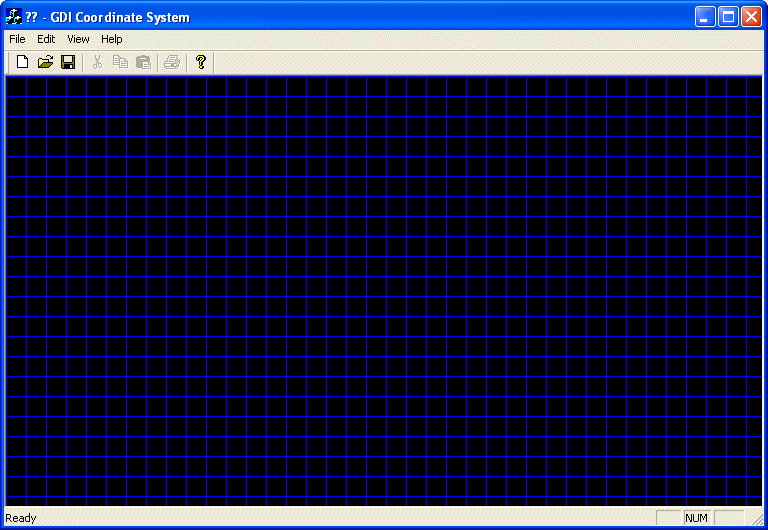Point Grid ```void CExoDraw1View::OnPaint() { CPaintDC dc(this); // device context for painting CRect Recto; GetClientRect(&Recto); CBrush bgBrush(BLACK_BRUSH); dc.SelectObject(bgBrush); dc.Rectangle(Recto); for(int x = 0; x < Recto.Width(); x += 20) { for(int y = 0; y < Recto.Height(); y += 20) { dc.SetPixel(x, y, RGB(255, 255, 255)); } } }```Sinus ```void CExoView::OnPaint() { CPaintDC dc(this); // device context for painting // TODO: Add your message handler code here dc.SetMapMode(MM_ANISOTROPIC); dc.SetViewportOrg(340, 220); dc.SetWindowExt(1440, 1440); dc.SetViewportExt(-1440, -220); CPen PenBlue(PS_SOLID, 1, RGB(0, 0, 255)); dc.SelectObject(PenBlue); // Axes dc.MoveTo(-300, 0); dc.LineTo( 300, 0); dc.MoveTo( 0, -1400); dc.LineTo( 0, 1400); // I am exaggerating with the PI value here but why not? const double PI = 3.141592653589793238462643383279; // The following two values were chosen randomly by me. // You can chose other values you like const int MultiplyEachUnitOnX = 50; const int MultiplyEachUnitOnY = 250; for(double i = -280; i < 280; i += 0.01) { double j = sin(PI / MultiplyEachUnitOnX * i) * MultiplyEachUnitOnY; dc.SetPixel(i, j, RGB(255, 0, 0)); } // Do not call CView::OnPaint() for painting messages }```